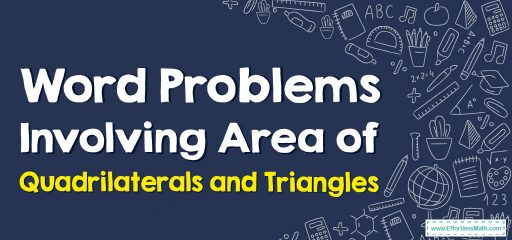# Word Problems Involving Area of Quadrilaterals and Triangles

In this article, the focus is on teaching you how to solve word problems involving the area of quadrilaterals and triangles.## A step-by-step guide to word problems involving the area of quadrilaterals and triangles

The word problem is a way of learning the area of quadrilaterals and triangles better.

The area of a parallelogram: $$A=base×height$$

The area of a triangle: $$A=\frac{1}{2}(base×height)$$

The area of a trapezoid: $$A= \frac{1}{2}×$$(Sum of parallel sides)$$×$$(perpendicular distance in between the parallel sides). $$\frac{1}{2}×(AB ̅+DC) ̅×(d)$$

### Word Problems Involving Area of Quadrilaterals and Triangles – Examples 1

Solve.
A triangle has an area of 64 square inches and a height of 16 inches. What is the length of the triangle’s base?
Solution:
Since the area of a triangle is $$\frac{1}{2}$$(base$$×$$height), then you must divide 64 by 16 and then multiply the product by 2 to find the length of its base.
$$64÷16=4$$
$$4×2=8$$
So, the length of the triangle’s base is 8 in.

### Word Problems Involving Area of Quadrilaterals and Triangles – Examples 2

Solve.
The area of a parallelogram is $$484 ft^2$$ and its height is 22 ft. What is the base length?
Solution:
Since the area of a parallelogram is base×height, so you have to divide 484 by 22 to find the base length.
$$484÷22=22$$
So, the base length of the parallelogram is 22 ft.

### What people say about "Word Problems Involving Area of Quadrilaterals and Triangles - Effortless Math: We Help Students Learn to LOVE Mathematics"?

No one replied yet.

X
30% OFF

Limited time only!

Save Over 30%

SAVE $5 It was$16.99 now it is \$11.99